# MCAT Physical : Continuity Equation

## Example Questions

### Example Question #1 : Continuity Equation

A liquid flows through a pipe with a diameter of 10cm at a velocity of 9cm/s. If the diameter of the pipe then decreases to 6cm, what is the new velocity of the liquid?

21cm/s

12cm/s

25cm/s

15cm/s

18cm/s

25cm/s

Explanation:

Rate of flow, A * v, must remain constant. Use the continuity equation,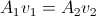.

Solving the initial cross-sectional area yields: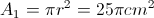. The initial radius is 5cm.

Then find the final area of the pipe: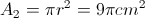. The final radius is 3cm.

Using these values in the continuity equation allows us to solve the final velocity.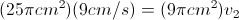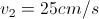### Example Question #2 : Continuity Equation

If blood flows through the aorta with velocity,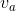, with what velocity would blood flow through the capillaries in the body?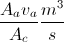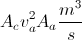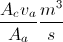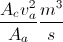Explanation:

Just like the volume flow rate equation for fluids, the flow rate of blood through the body is equal to area times velocity.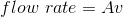The flow rate is a constant, so depending on the area that the blood is travelling through, the velocity is constantly changing; therefore the volume flow rate though the aorta is equal to the volume flow rate in the capillaries.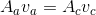Because we can determine the area of the aorta and area of the capillaries, knowing the velocity through the aorta can give the velocity through the capillaries.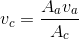### Example Question #3 : Continuity Equation

Which will produce the greatest increase in flow velocity through a tube?

Doubling the tube area

Dividing the tube area by three

Doubling the viscosity of the liquid

Explanation:

The equation for volumetric flow rate is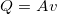, whereis the cross-sectional area of the tube andis the flow velocity. We can re-write this equation to solve for the velocity and include the tube radius.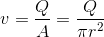Halving the radius will reduce the tube area by a factor of four.

Volumetric flow rate is constant, thus, any reduction in area will cause a corresponding increase in velocity.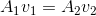Halving the radius will thus quadruple the velocity, resulting in the greatest increase of the given options.

### Example Question #81 : Fluids And Gases

A pipe with a diameter of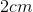is attached to a garden hose with a nozzle. If the velocity of flow in the pipe is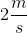, what is the velocity of the flow at the nozzle when it is adjusted to have a diameter of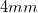?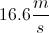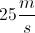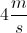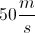Explanation:

Flow rate in a pipe must be constant in order to create linear flow. This flow rate is given by the product of the cross-sectional area and the velocity of the fluid.The cross-sectional areas of the pipe and nozzle can be found using their radii.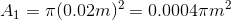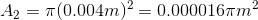Use these areas and the initial velocity to calculate the final velocity in the nozzle.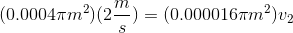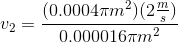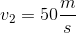### Example Question #1 : Continuity Equation

If a pipe with flowing water has a cross-sectional area nine times greater at point 2 than at point 1, what would be the relation of flow speed at the two points?

The flow speed relation will depend on the viscosity of the water

The flow speed at point 2 is three times that at point 1

The flow speed at point 1 is three times that at point 2

The flow speed at point 1 is nine times that at point 2

The flow speed at point 2 is nine times that at point 1

The flow speed at point 1 is nine times that at point 2

Explanation:

Using the continuity equation we know that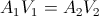. The question tells us that the cross-sectional area at point 2 is nine times greater that at point 1 (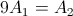).

Using the continuity equation we can make A1= 1 and A2 = 9.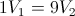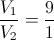Flow speed at point 1 is nine times that at point 2.

### Example Question #6 : Continuity Equation

As water is traveling from a water tower, to somone's home, the pipes it travels in frequently change size.

Water is traveling at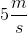in a tube with a diameter of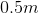. The tube gradually increases in size to a diameter of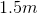, and then gradually decreases to a diameter of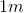. Neglecting any energy losses due to friction and pressure changes, what is the speed of the water when it reaches the tube diameter of?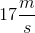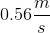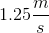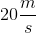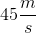Explanation:

This problem covers the concept of continuity. As the tube diameter changes, the volumetric flow of water stays constant. Therefore, we can calculate the volumetric flow at the diameter of 0.5m, and use that to find the velocity of water at 1m.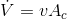Here,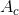is the cross-sectional area of the pipe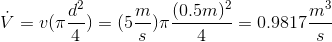Apply this flow rate to a tube diameter of 1m to find the velocity: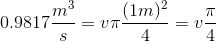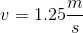Alternatively, this question can be solved by setting up a proportion.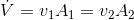Rearranging for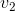: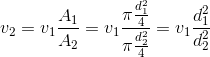Plugging in our values: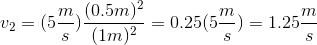### Example Question #1 : Continuity Equation

Blood travels through an artery at velocity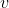. If a vasoconstricting chemical is consumed and the artery constricts to half the original diameter, what is the new velocity of the blood?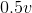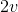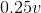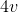Explanation:

The continuity equation states that:In other words, the volumetric flow rate stays constant throughout a pipe of varying diameter. If the diameter decreases (constricts), then the velocity must increase.

To establish the change in cross-sectional area, we need to find the area in terms of the diameter: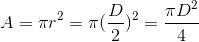If the diameter is halved, the area is quartered.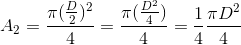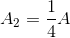To keep the volumetric flow constant, the velocity would have to increase by a factor of 4.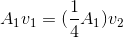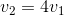### All MCAT Physical Resources